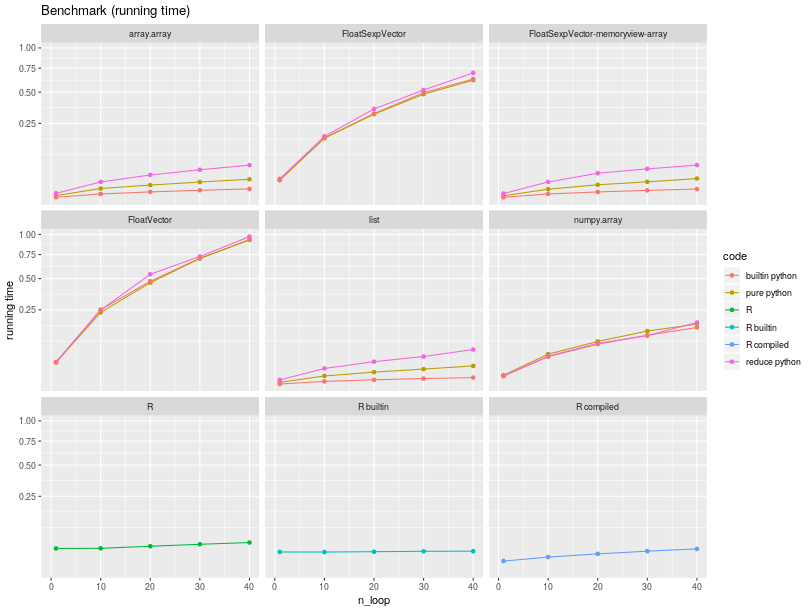# Performances¶

## Optimizing for performances¶

### Memory usage¶

R objects live in the R memory space, their size unbeknown to Python, and because of that it seems that Python does not always garbage collect often enough when large objects are involved. This is sometimes leading to transient increased memory usage when large objects are overwritten in loops, and although reaching a system’s memory limit appears to trigger garbage collection, one may wish to explicitly trigger the collection.

```import gc
gc.collect()
```

As a concrete example, consider the code below. This has been used somewhere a unique benchmark Python-to-R bridge, unfortunately without considering specificities of the Python and R respective garbage collection mechanisms. The outcome of the benchmark changes dramatically, probably putting back rpy2 as the fastest, most memory efficient, and most versatile Python-to-R bridge.

```import rpy2.robjects
import gc

r = rpy2.robjects.r

r("a <- NULL")
for i in range(20):
rcode = "a <- rbind(a, seq(1000000) * 1.0 * %d)" % i
r(rcode)
print r("sum(a)")
# explicit garbage collection
gc.collect()
```

### Low-level interface¶

The high-level layer `rpy2.robjects` brings a lot of convenience, such a class mappings and interfaces, but obviously with a cost in term of performances. This cost is believe to be neglibible for common use cases (calling calling complex R code in libraries with no Python alternative or with comparable level of maturity), but compute-intensive programms traversing the Python-to-R bridge way and back a very large number of time will notice it.

The `rpy2.rinterface` low-level layer gets the programmer closer to R’s C-level interface, but when interfacing with R using `cffi`’s ABI mode this does not translate into immediate noticeable speed gains. However, having code for the `rpy2.rinterface` interface means that translation to C is relatively easy to achieve, and `cffi`’s API mode can be then used.

Note

General speed improvement strategies for Python will apply. For example `cython` can compile to C Python-like code with type declarations or pypy can be used as an alternative implemenation of Python.

When the compute-intensive shuttling across Python and R is mainly about Python accessing data in R data structures, a memoryview (available as `rpy2.rinterface.BoolSexpVector.memoryview()`, `rpy2.rinterface.FloatSexpVector.memoryview()`, or `rpy2.rinterface.IntSexpVector.memoryview()`) will provide access to the memory region in the embedded R where data for an array is stored. The numpy array interface as `rpy2.rinterface.NumpyArrayInterface.__array_interface__` for the same vector objects.

## A naive benchmark¶

As a naive benchmark, we took a function that would sum up all elements in a numerical vector.

In pure R, the code is like:

```function(x)
{
total = 0;
for (elt in x) {
total <- total + elt
}
}
```

while in pure Python this is like:

```def python_sum(x):
total = 0.0
for elt in x:
total += elt
```

R has obviously a vectorized function sum() calling underlying C code, but the purpose of the benchmark is to measure the running time of pure R code.

We ran this function over different types of sequences (of the same length)

```    n = 20000
x_list = [random.random() for i in range(n)]
module = None
if kind == "array.array":
import array as module
res = module.array('f', x_list)
elif kind == "numpy.array":
import numpy as module
res = module.array(x_list, 'f')
elif kind == "FloatVector":
import rpy2.robjects as module
res = module.FloatVector(x_list)
elif kind == "FloatSexpVector":
import rpy2.rinterface as module
module.initr()
res = module.FloatSexpVector(x_list)
elif kind == "FloatSexpVector-memoryview-array":
import rpy2.rinterface as module
module.initr()
tmp = module.FloatSexpVector(x_list)
mv = tmp.memoryview()
res = array.array(mv.format, mv)
elif kind == "list":
res = x_list
elif kind == "R":
import rpy2.robjects as module
res = module.rinterface.FloatSexpVector(x_list)
module.globalenv['x'] = res
res = None
```

The running times are summarized in the figure below.Iterating through a `list` is the fastest, explaining why implementations of the sum in pure Python over a `list` of numbers is the fastest. Python is much faster than R for iterating through a vector/list (almost 9 times faster in this run).

Measuring the respective slopes, and using the slope for the R code as reference we obtain relative speedup, that is how many times faster code runs.

Function

Sequence

Speedup

builtin python

array.array

3.40

builtin python

FloatSexpVector

0.02

builtin python

FloatSexpVector-memoryview-array

3.55

builtin python

FloatVector

0.02

builtin python

list

5.62

builtin python

numpy.array

0.10

pure python

array.array

0.90

pure python

FloatSexpVector

0.02

pure python

FloatSexpVector-memoryview-array

0.83

pure python

FloatVector

0.02

pure python

list

0.91

pure python

numpy.array

0.09

R builtin

R builtin

8.78

R compiled

R compiled

0.81

R

R

1.00

reduce python

array.array

0.30

reduce python

FloatSexpVector

0.02

reduce python

FloatSexpVector-memoryview-array

0.29

reduce python

FloatVector

0.02

reduce python

list

0.27

reduce python

numpy.array

0.09

The object one iterates through matters much for the speed, and the poorest performers are our `rpy2.robjects.vectors.FloatVector` and `rpy2.rinterface.FloatSexpVector` (50 times slower than pure R in this run). Relatively unimpressive performance is expected since the iteration calls for each element in the R vector pure-Python code that performs various calling C for the extraction the element corresponding to the index.

On the other hand, exposing the content of the R vector through a memoryview and `array.array` leads to a rather nice speedup by letting us operate at the same level of performance as if it was a Python array. In other words, rpy2 can make computations on R vectors using Python faster than if using R itself. R bridges relying on pipes or client-server architectures (e.g., RServe) will not be able to offer such performance.

What might seem more of a surprise is that iterating through a `numpy.array` is quite slower than pure R (10 times slower no less). This is happening the parsing of the argument is not as streamlined, and not as much straightforward C, as in R.

Finally, and to put the earlier benchmarks in perspective, it would be fair to note that python and R have a builtin function sum, calling C-compiled code. This is just a synthetic example to illustrate a point about data in memory regions and code to access that data, not intended to represent a general assessment of expected performances.